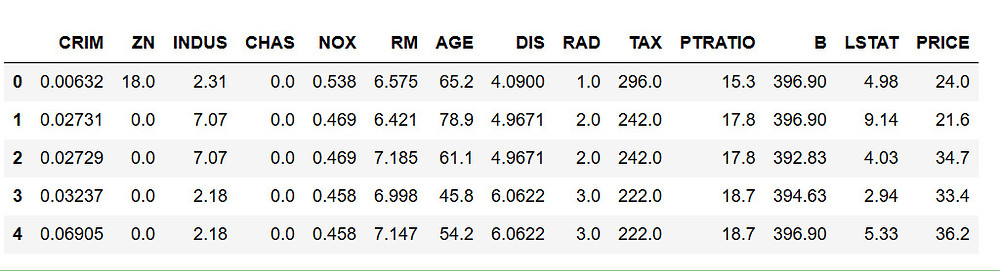Search

# Predict Boston House Prices Using Python & Linear Regression

In machine learning, the ability of a model to predict continuous or real values based on a training dataset is called Regression. With a small dataset and some great python libraries, we can solve such a problem with ease.

In this blog post, we will learn how to solve a supervised regression problem using the famous Boston housing price dataset. Other than location and square footage, a house value is determined by various other factors. Let’s analyze this problem in detail and using machine learning model to predict a housing price.Dependencies

• pandas - To work with solid data-structures, n-dimensional matrices and perform exploratory data analysis.

• matplotlib - To visualize data using 2D plots.

• seaborn - To make 2D plots look pretty and readable.

• scikit-learn - To create machine learning models easily and make predictions.

Boston Housing Prices Dataset

In this dataset, each row describes a boston town. There are 506 rows and 13 attributes (features) with a target column (price).

The problem that we are going to solve here is that given a set of features that describe a house in Boston, our machine learning model must predict the house price. To train our machine learning model with boston housing data, we will be using scikit- learn’s boston dataset.

We will use pandas and scikit-learn to load and explore the dataset. The dataset can easily be loaded from scikit-learn datasets module using load_boston function

```import pandas as pd
from sklearn import datasets

There are four keys in this dataset using which we can access more information about the dataset .["data ", "target", "feature_name" and "DESCR"] are the four keys which could be accessed using keys() on the dataset variable.To know the description of each column name in this dataset, we can use DESCR to display the description of this dataset .Exploratory Data Analysis histogram plot

We can easily convert the dataset into a pandas dataframe to perform exploratory data analysis. Simply pass in the boston.data as an argument to pd.DataFrame(). We can view the first 5 rows in the dataset using head() function.

```bos = pd.DataFrame(boston.data, columns = boston.feature_names)
bos['PRICE'] = boston.targetExploratory Data Analysis is a very important step before training the model. Here, we will use visualizations to understand the relationship of the target variable with other features.

Let’s first plot the distribution of the target variable. We will use the histogram plot function from the matplotlib library.